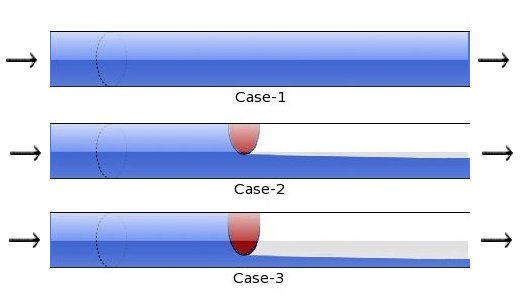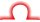black nike shoes roshe cheapnicesports.com

# Resistors

Resistor is a component that limits the flow of current. It is similar to the blockage in a pipe carrying water. It limits the flow of water. In many electronics applications we need to limit the current and this is acheived by putting resistor in current's path.The above figure shows three cases of a pipe carrying water.

Case I : In case one, water is flowing smoothly through the pipe since there is no blockage. In electronics this condition is same as a wire carrying current, where current is flowing smoothly since there is no electrical blockage in the path.

Case II : In case 2, a small blockage is introduced in the pipe and due to this blockage the water flow is reduced. This situation is similar where current flow is reduced by putting a small resistor in the path.

Case III : Case 3 shows that the blockage is further increased, which is allowing very less water to flow now. This is like putting a big resistor which allows very small current to flow through wire.

From the above analysis we can conclude following points :-

1. Resistor reduces the flow of current by providing blockage.
2. More Blockage will result into less flow of current.
3. These blockages in electronics domain are measured in ohms, more ohms means more blockage. Hence all resistor values in electronics are measured in ohms.
4. Symbolically ohms is represented by